gdix

•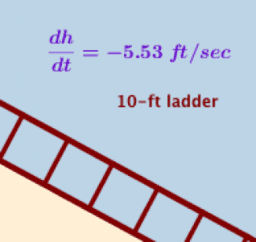Related Rates

Book

gdix

•Puzzle and fractions. Who Am I?

Activity

gdix

•Puzzle. Who Am I?

Activity

gdix

•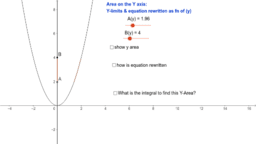Integration and Area on Y axis with Y Limits

Activity

gdix

•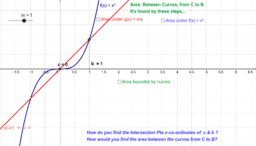Integration and Area between 2 curves Introduction

Activity

gdix

•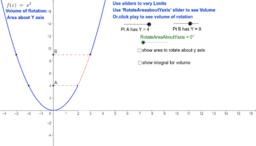Integration & Volume of solids by Rotation about the Y axis

Activity

gdix

•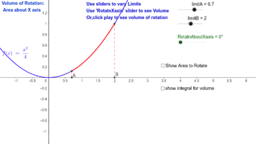Integration and Volume of solids of rotation

Activity

gdix

•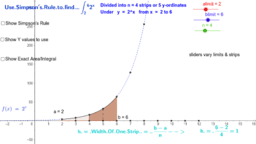Integration Approximate method using Simpsons rule

Activity

gdix

•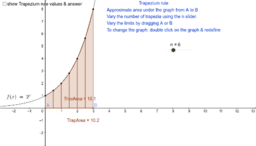Integration approximate method using Trapezia for area

Activity

gdix

•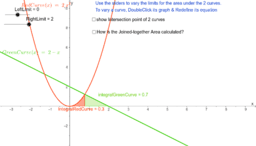Integration and joined Areas under 2 curves

Activity

gdix

•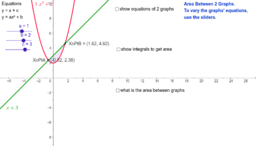Integration and Area between 2 curves

Activity

gdix

•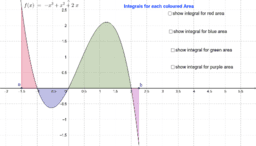Integration and Area above and below x axis (2)

Activity

gdix

•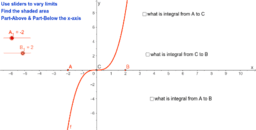Integration and Area above & below the X axis

Activity

gdix

•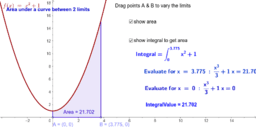Integration Process for finding Area

Activity

gdix

•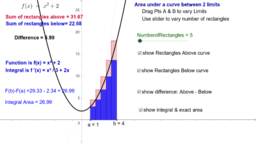Integration Area & Rectangles above and below Curve

Activity

gdix

•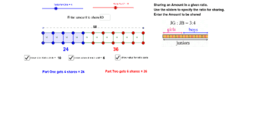Ratio Problems. Sharing an amount

Activity

gdix

•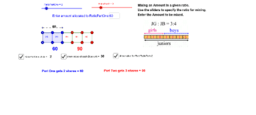Ratio Problems. Given the amount for one part.

Activity

gdix

•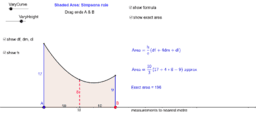Simpson's Rule for calculating areas with a curved side

Activity

gdix

•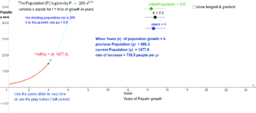Exponential growth of population

Activity

gdix

•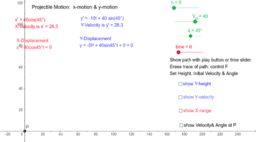Projectile Motion & Equations

Activity

gdix

•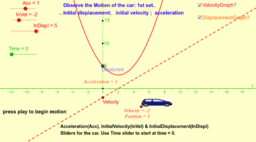Straight line motion and calculus 2

Activity

gdix

•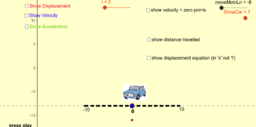Straight line motion and calculus

Activity

gdix

•Activity

gdix